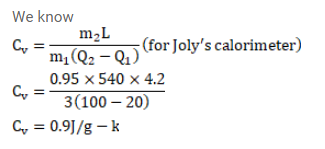# In Joly's differential steam calorimeter,Question:

In Joly's differential steam calorimeter, $3 \mathrm{~g}$ of an ideal gas is contained in a rigid closed sphere at $20^{\circ} \mathrm{c}$. The sphere is heated by steam at $100^{\circ} \mathrm{C}$ and it is found that an extra $0.095 \mathrm{~g}$ steam has condensed into water as the temperature of the gas becomes constant. Calculate the specific heat capacity of the gas in $\mathrm{J} / \mathrm{g}-\mathrm{K}$. The latent heat of vaporization of water $=540 \mathrm{cal} / \mathrm{g}$.

Solution: